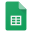# Methodology

## The Better Place Index

model and composition

So-called linear regression models allow us to measure the statistical effect of particular independent variables on the dependent variables, for example GDP per Capita and the Better Place Index. Based on the correlations above we have established statistical associations.

However, from a policy-making perspective, we are not merely interested in the correlations. But also how much, for example, a one percent increase in the marginal tax rate is required for an increase in the average income. This can be done by using a linear regression model, in particular so-called B-coefficients.TBPI Regression Model